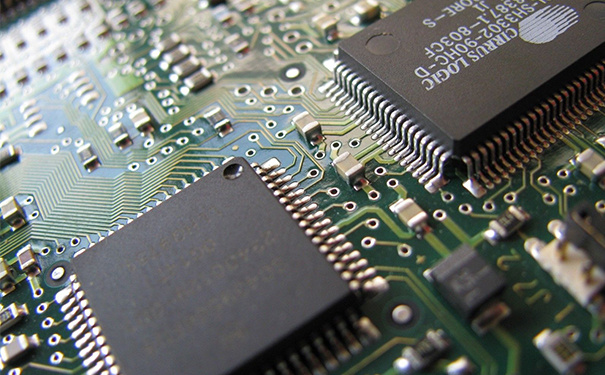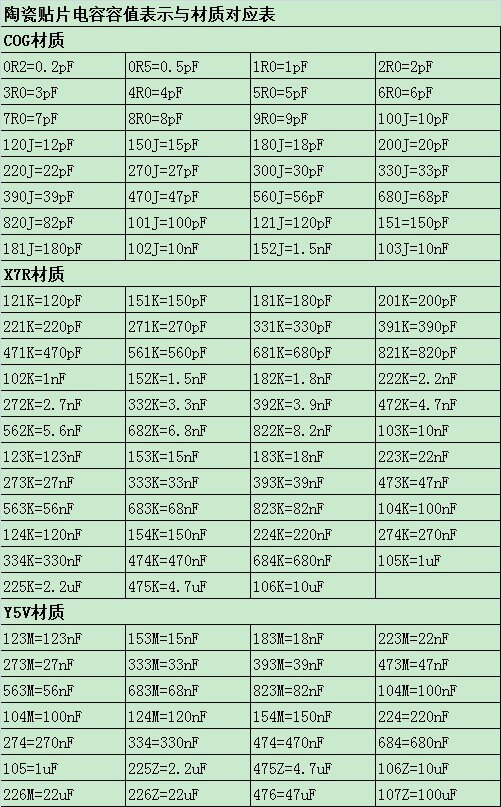# 电容容值如何表示？换算单位方法是什么？

1F(法拉)=1000mF(毫法) ，1(毫法) =1000uF(微法) ，1(微法) =1000nF(纳法) ，1(纳法)=1000pF(皮法)。

101(pF)=10 * 10^1 = 100pF = 0.1nF；

104(pF)=10 * 10^4 = 100000pF = 0.1uF；

105(pF)=10 * 10^5 = 1000000pF = 1uF。1uf=1000nf=1000000pf

1F(法拉)=1000mF(毫法)，1mF(毫法)=1000uF(微法)，1uF(微法)=1000nF(纳法)，1nF(纳法)=1000pF(皮法)。

101(pF)=10*10^1=100pF=0.1nF；

104(pF)=10*10^4=100000pF=0.1uF；

105(pF)=10*10^5=1000000pF=1uF。

1F=1000Mf=1000000μf=1000000000nf=1000000000000Pf1μf=1000nf=1000000pF1nf=1000pf### 关注我们